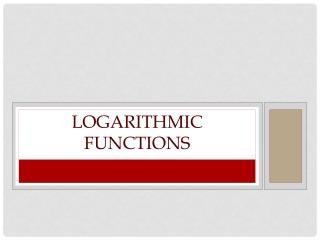DownloadDownload PresentationLogarithmic Functions

# Logarithmic Functions

Télécharger la présentation## Logarithmic Functions

- - - - - - - - - - - - - - - - - - - - - - - - - - - E N D - - - - - - - - - - - - - - - - - - - - - - - - - - -
##### Presentation Transcript

1. Logarithmic Functions

2. Log Functions • Changing Forms • y = logbx x = by • Use these formulas to help you rewrite log functions. • We rewrite them so they are in forms that are easier to work with. • log28 = 3 • log93 = • log5() = -2 8 = 23 3 = 91/2 = 5-2 These actually make sense, mathematically!

3. Log Functions • You should be able to go both ways! • Remember: • y = logbx x = by • Try these: • log39 = 2 log164 = log2() = -3 • 9 = 32 4 = 161/2 = 2-3 • 81 = 92 12 = = 7-2 • log981 = 2 log14412 = log7() = -2

4. Log Functions • Exact Values • Find the exact value of log381 • log381 = x • 3x = 81 • 3x = 34 • x = 4 • Set the equation equal to “x”. • Can’t solve problems without a variable. • Rewrite in exponential form. • That is why we did all that! • Rewrite “81” so it looks the same as the other side. • Make 81 look like 3 • Set the exponents equal to each other.

5. Log Functions • I know that is a bit tricky. Let’s try again. • Find the exact value of log16913. • log16913 = x • 169x = 13 • (132)x = 13 • 132x = 13 • 2x = 1 • x = 1/2 • Set the equation equal to “x”. • Can’t solve problems without a variable. • Rewrite in exponential form. • That is why we did all that! • Rewrite “169” so it looks the same as the other side. • Wait that is not the same as last time!! Why? • Set the exponents equal to each other.

6. Log Functions • Try a few more to make sure you know what to do. • log5() • log2() • log10010 • log101000 x = -1 x = -1 x = ½ x = 3